Question
A 2.5 ampere current flows through a wire for 5.0 minutes. (a) How many coulombs of charge flow through the wire in this time? (b) How many electrons flow through the wire in this time?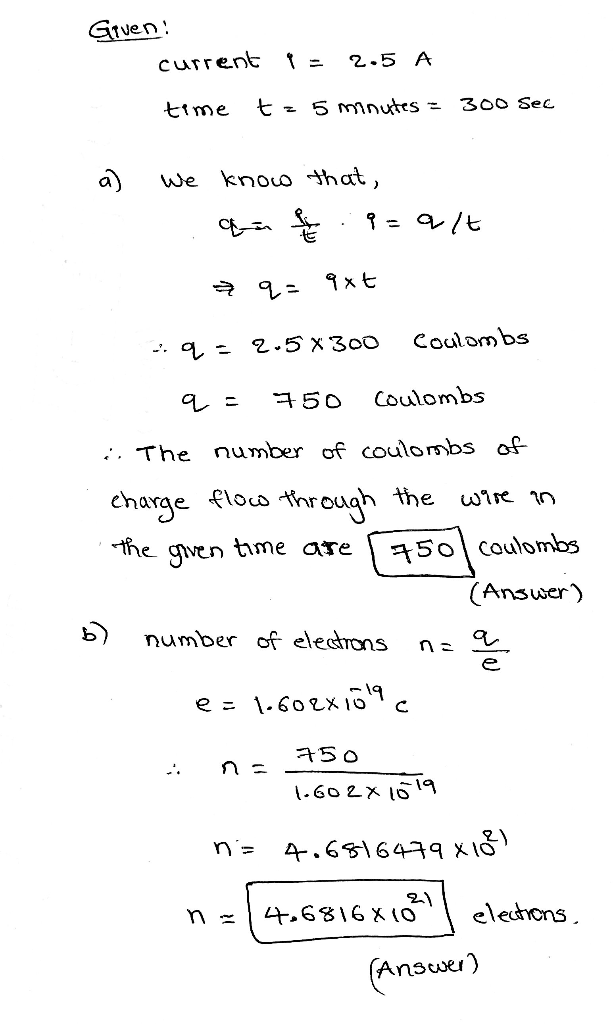#### Earn Coins

Coins can be redeemed for fabulous gifts.

Similar Homework Help Questions
• ### 43. If a 2.5 A current flows through a circuit for 35 minutes, how many coulombs...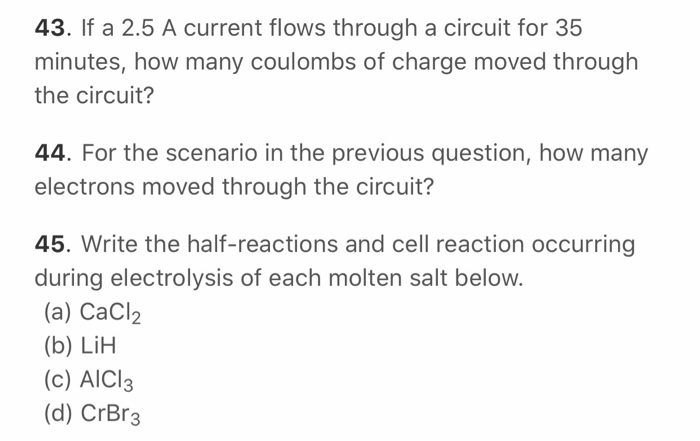43. If a 2.5 A current flows through a circuit for 35 minutes, how many coulombs of charge moved through the circuit? 44. For the scenario in the previous question, how many electrons moved through the circuit? 45. Write the half-reactions and cell reaction occurring during electrolysis of each molten salt below. (a) CaCl2 (b) LIH (c) AICI3 (d) CrBr3

• ### 11. If a 2.5 A current flows through a circuit for 40 s, how many coulombs...11. If a 2.5 A current flows through a circuit for 40 s, how many coulombs of charge moved through the circuit? 12. Consider two processes: sublimation of Iz(s) and melting of 12(s) at the same temperature (Note: the latter process can occur at the same temperature but somewhat higher pressure). (A) 12(s)I(9) and (B) 12(5) I2(1) Is AS positive or negative in these processes? In which of the processes will the magnitude of the entropy change be greater? -...

• ### A 2.50 A current flows through the leads of an electric appliance. How many coulombs pass...

A 2.50 A current flows through the leads of an electric appliance. How many coulombs pass through it in 5.0 min?

• ### Suppose a current of 0.810 A flows through a copper wire for 30.0 minutes. Calculate how...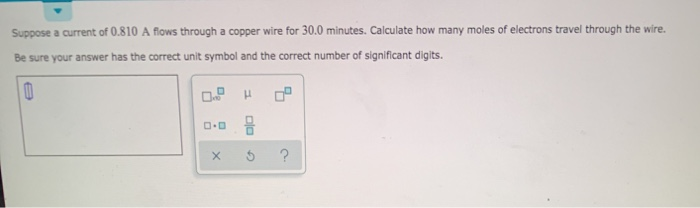Suppose a current of 0.810 A flows through a copper wire for 30.0 minutes. Calculate how many moles of electrons travel through the wire. Be sure your answer has the correct unit symbol and the correct number of significant digits.

• ### A 10-A current flows for two minutes. (a) How much charge has passed through this circuit?...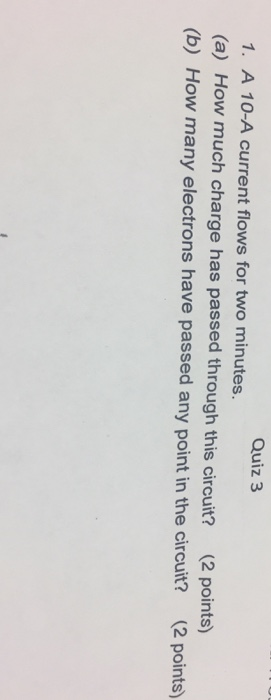A 10-A current flows for two minutes. (a) How much charge has passed through this circuit? (b) How many electrons have passed any point in the circuit?

• ### answer all parts ELECTRICAL EQUATIONS: Current (A) = Charge (C) / Time (5) | Energy (1) = Potential Difference (V)...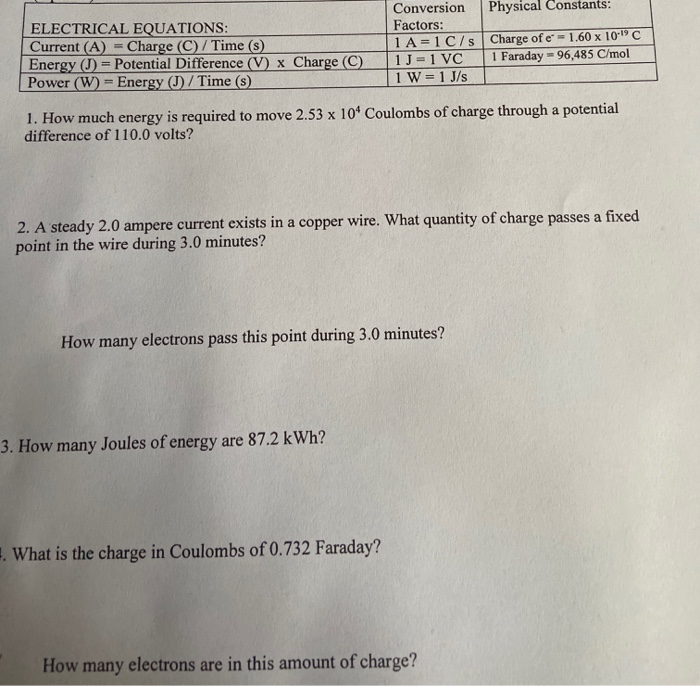answer all parts ELECTRICAL EQUATIONS: Current (A) = Charge (C) / Time (5) | Energy (1) = Potential Difference (V) x Charge C Power (W) = Energy D / Time (s) Conversion Physical Constants: Factors: 1A=1C/s Charge of e- 1.60 x 10°C 1 -1 VC Faraday - 96,485 C/mol 1W= 1 J/s 1. How much energy is required to move 2.53 x 104 Coulombs of charge through a potential difference of 110.0 volts? 2. A steady 2.0 ampere current exists...

• ### There is a current of 10 A in a 100 W resistor. (a) How many coulombs...

There is a current of 10 A in a 100 W resistor. (a) How many coulombs of charge and (b) how many electrons passed through any cross-section of the resistor in 1-minute?

• ### QUESTION 6 The allowed safe current for a No. 3 copper wire, 0.2294 inch in diameter,...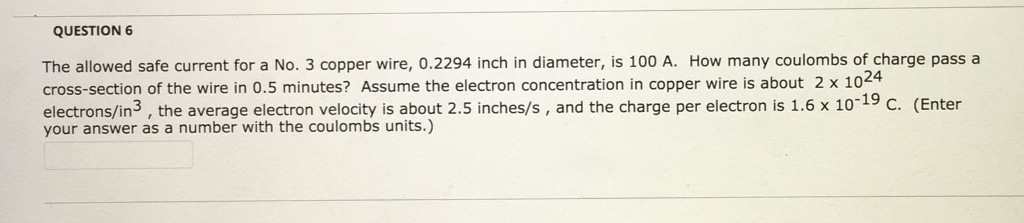QUESTION 6 The allowed safe current for a No. 3 copper wire, 0.2294 inch in diameter, is 100 A. How many coulombs of charge pass a cross-section of the wire in 0.5 minutes? Assume the electron concentration in copper wire is about 2 x 102④ electrons/in3, the average electron velocity is about 2.5 inches/s, and the charge per electron is 1.6x 1019 C. (Enter your answer as a number with the coulombs units.)

• ### A steady electric current flows through a wire. If 8.0 C of charge passes a particular...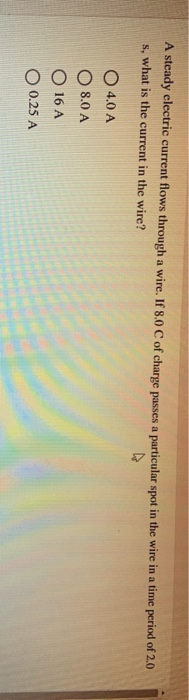A steady electric current flows through a wire. If 8.0 C of charge passes a particular spot in the wire in a time period of 2.0 s, what is the current in the wire? O 4.0 A O 8.0 A O 16 A O 0.25 A If the current is a constant 5.0 A, how long will it take for 14.0 C of charge to move past a particular spot in the wire? O 70 s O 14,0 s O...

• ### QUESTION 1 The allowed safe current for a No. 8 copper wire, 0.1285 inch in diameter,...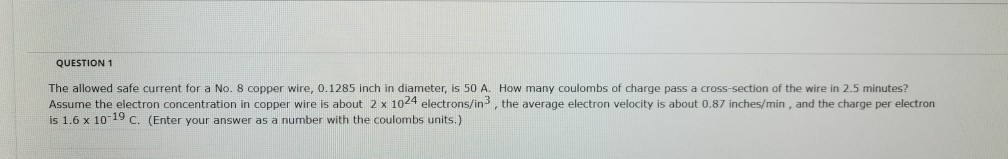QUESTION 1 The allowed safe current for a No. 8 copper wire, 0.1285 inch in diameter, is 50 A. How many coulombs of charge pass a cross-section of the wire in 2.5 minutes? is 1.6 x 10-19 C. (Enter your answer as a number with the coulornbs units.) Assume the electron concentration in copper wire is about 2 x 1024 electrons/in3, the average electron velocity is about 0.87 inches/min, and the charge per electron